# Why to use extract() function in PHP ?

• Last Updated : 10 Mar, 2022

Extract() function is an inbuilt function in PHP which is used when there is conflict in the variable name. When someone writes a code of approximately 100 lines, there is a chance that he declares the variable already then there is a conflict between what value the variable we assign. By using the extract() function we can choose which value to be saved.

Also, we can use it to extract the variable of an array into the symbol table. It extracts key-value pairs in different symbols and we can access them directly by calling their variable. Always remember that it can not be used for the user-given data where we can take data from some get or post or any other methods.

` extract(array, extract_rules, prefix)`
• array: We can pass the array in which we want to extract.
• extract_rules: There are 8 types of rules which perform different types of things
1. EXTR_OVERWRITE
2. EXTR_SKIP
3. EXTR_IF_EXISTS
4. EXTR_REFS
5. EXTR_PREFIX_SAME
6. EXTR_PREFIX_INVALID
7. EXTR_PREFIX_IF_EXISTS
8. EXTR_PREFIX_ALL
• prefix: This declares the variable by a prefix along with underscore. It is only used when rules are EXTR_PREFIX_SAME, EXTR_PREFIX_ALL,       EXTR_PREFIX_INVALID or EXTR_PREFIX_IF_EXISTS

We can understand every rule one by one by the given examples.

Example 1: In this, we simply pass the array and there are no extract rules. So it can do nothing with \$a and it remains unchanged.

## PHP

 ` ``"Geeks"``,``"b"` `=> ``"for"``, ``"c"` `=> ``"Geeks"``);``    ``extract(``\$my_array``);``    ``echo` `"a = \$a "``.``"
"``; ``    ``echo` `"b = \$b "``.``"
"``;``    ``echo` `"c = \$c "``;``     ` `?>`

Output: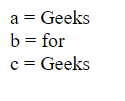Example 2: In this, we use EXTR_OVERWRITE, so it overwrites the variable value. Also, there is no prefix used as mentioned above.

## PHP

 ` ``"Geeks"``,``"b"` `=> ``"for"``, ``"c"` `=> ``"Geeks"``);``    ``extract(``\$my_array``, EXTR_OVERWRITE);``    ``echo` `"a = \$a "``.``"
"``; ``    ``echo` `"b = \$b "``.``"
"``;``    ``echo` `"c = \$c "``.``"
"``;`` ` `?>`

Output:Example 3: In this, we use EXTR_SKIP, so it skips the value after assigning the variable as you can see that \$a value retained its value and print “GeeksforGeeks”. There is no prefix used as mentioned above.

## PHP

 ` ``"Geeks"``,``"b"` `=> ``"for"``, ``"c"` `=> ``"Geeks"``);``    ``extract(``\$my_array``, EXTR_SKIP);``    ``echo` `"a = \$a "``.``"
"``; ``    ``echo` `"b = \$b "``.``"
"``;``    ``echo` `"c = \$c "``.``"
"``;`` ` `?>`

Output: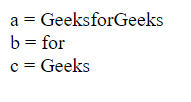Example 4: In this, we use EXTR_IF_EXISTS, so it retained the variable which is already declared and otherwise does nothing to a non-matched variable. As you can see in the below image there is undefined variable b,c because it is not already declared, so they are not extracted.

## PHP

 ` ``"Geeks"``,``"b"` `=> ``"for"``, ``"c"` `=> ``"Geeks"``);``    ``extract(``\$my_array``, EXTR_IF_EXISTS);``    ``echo` `"a = \$a "``.``"
"``; ``    ``echo` `"b = \$b "``.``"
"``;``    ``echo` `"c = \$c "``.``"
"``;`` ` `?>`

Output: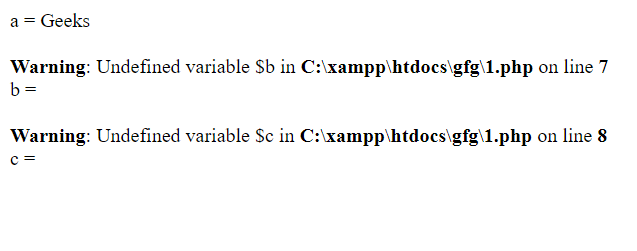Example 5: In this, we use EXTR_REFS, so it refers to the same variable after extracting it. As you can see, you can access all the variables just by their value name as shown in the below image.

## PHP

 ` ``"Geeks"``,``"b"` `=> ``"for"``, ``"c"` `=> ``"Geeks"``);``    ``extract(``\$my_array``, EXTR_REFS);``    ``echo` `"a = \$a "``.``"
"``; ``    ``echo` `"b = \$b "``.``"
"``;``    ``echo` `"c = \$c "``.``"
"``;`` ` `?>`

Output:Example 6: In this, we use EXTR_PREFIX_SAME. In this when the variable is already declared then it retained its value and it creates the new variable with prefix along with underscore variable and then assigns the extracted value to it as shown in the below image.

## PHP

 ` ``"Geeks"``,``"b"` `=> ``"for"``, ``"c"` `=> ``"Geeks"``);``    ``extract(``\$my_array``, EXTR_PREFIX_SAME,``"gfg"``);``    ``echo` `"a = \$a "``.``"
"``; ``    ``echo` `"b = \$b "``.``"
"``;``    ``echo` `"c = \$c "``.``"
"``;``    ``echo` `"gfg_a = \$gfg_a "``.``"
"``;`` ` `?>`

Output: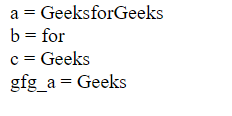Example 7: In this, we use EXTR_PREFIX_INVALID. There is an array with numerical keys and when we extract the value it creates variables with prefix name and assigns the values as shown below image.

## PHP

 `"``;``    ``echo` `"gfg_1 = \$gfg_1 "``.``"
"``;``    ``echo` `"gfg_2 = \$gfg_2 "``.``"
"``;`` ` `?>`

Output: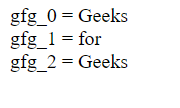Example 8: In this, we use EXTR_PREFIX_IF_EXISTS, if the variable is already declared then it retained its value and assign the new variable with prefix and assign extract value as shown in the below image. It only creates a prefix variable if a non-prefixed version of the same variable exists.

## PHP

 ` ``"Geeks"``,``"b"` `=> ``"for"``, ``"c"` `=> ``"Geeks"``);``    ``extract(``\$my_array``, EXTR_PREFIX_IF_EXISTS,``"gfg"``);``    ``echo` `"a = \$a "``.``"
"``; ``    ``echo` `"b = \$b "``.``"
"``;``    ``echo` `"c = \$c "``.``"
"``;``    ``echo` `"gfg_a = \$gfg_a "``.``"
"``;`` ` `?>`

Output: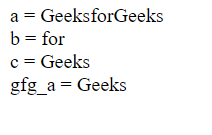Example 9: In this, we use EXTR_PREFIX_ALL, so it extracts all variables with prefix names and assigns the value and the original variable remains unchanged.

## PHP

 ` ``"Geeks"``,``"b"` `=> ``"for"``, ``"c"` `=> ``"Geeks"``);``    ``extract(``\$my_array``, EXTR_PREFIX_ALL,``"gfg"``);``    ``echo` `"a = \$a "``.``"
"``; ``    ``echo` `"gfg_a = \$gfg_a "``.``"
"``;``    ``echo` `"gfg_b = \$gfg_b "``.``"
"``;``    ``echo` `"gfg_c = \$gfg_c "``.``"
"``;`` ` `?>`

Output: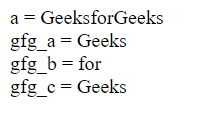My Personal Notes arrow_drop_up